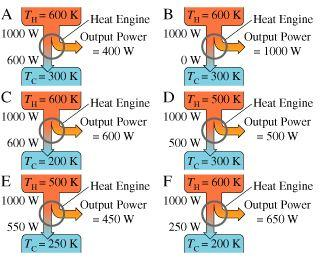# Problem: Which of the remaining designs has the highest thermal efficiency?

###### FREE Expert Solution

Efficiency:

$\overline{){\mathbf{\eta }}{\mathbf{=}}\frac{{\mathbf{W}}_{\mathbf{o}\mathbf{u}\mathbf{t}}}{{\mathbf{W}}_{\mathbf{i}\mathbf{n}}}}$

ηA = 400W/1000W = 0.4

ηB = 1000W/1000W = 1

89% (171 ratings)###### Problem Details

Which of the remaining designs has the highest thermal efficiency?